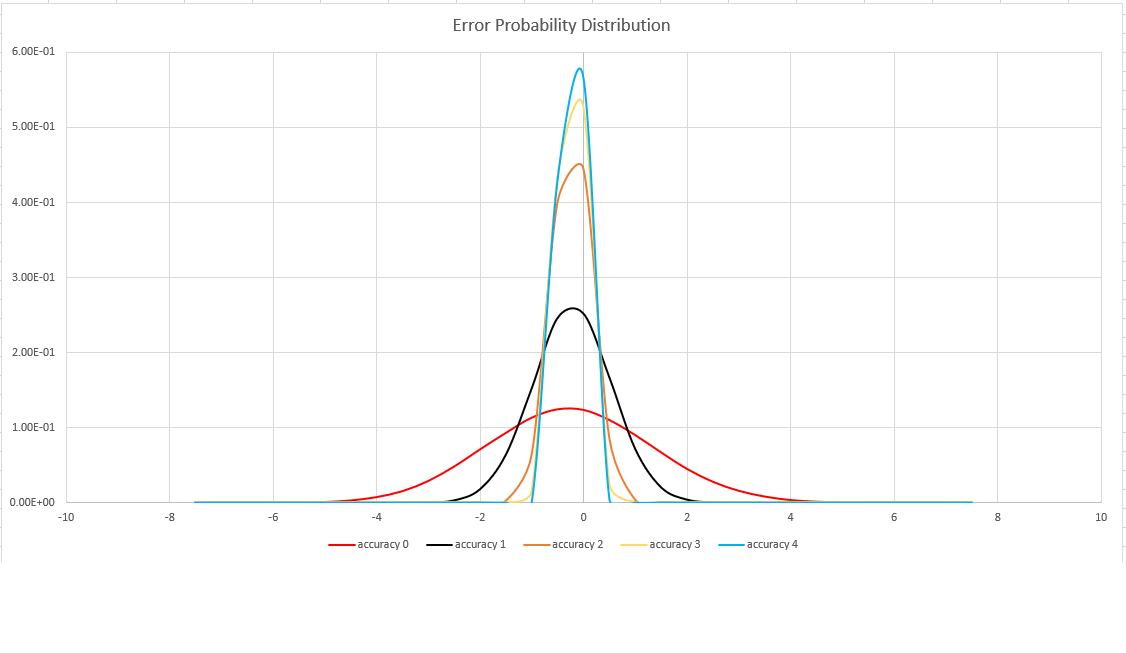# dcount() (aggregation function)

Calculates an estimate of the number of distinct values that are taken by a scalar expression in the summary group.

Null values are ignored and don't factor into the calculation.

Note

The `dcount()` aggregation function is primarily useful for estimating the cardinality of huge sets. It trades accuracy for performance, and may return a result that varies between executions. The order of inputs may have an effect on its output.

Note

This function is used in conjunction with the summarize operator.

## Syntax

`dcount` `(`expr[`,` accuracy]`)`

## Parameters

Name Type Required Description
expr string The input whose distinct values are to be counted.
accuracy int The value that defines the requested estimation accuracy. The default value is `1`. See Estimation accuracy for supported values.

## Returns

Returns an estimate of the number of distinct values of expr in the group.

## Example

This example shows how many types of storm events happened in each state.

``````StormEvents
| summarize DifferentEvents=dcount(EventType) by State
| order by DifferentEvents
``````

The results table shown includes only the first 10 rows.

State DifferentEvents
TEXAS 27
CALIFORNIA 26
PENNSYLVANIA 25
GEORGIA 24
ILLINOIS 23
MARYLAND 23
NORTH CAROLINA 23
MICHIGAN 22
FLORIDA 22
OREGON 21
KANSAS 21
... ...

## Estimation accuracy

This function uses a variant of the HyperLogLog (HLL) algorithm, which does a stochastic estimation of set cardinality. The algorithm provides a "knob" that can be used to balance accuracy and execution time per memory size:

Accuracy Error (%) Entry count
0 1.6 212
1 0.8 214
2 0.4 216
3 0.28 217
4 0.2 218

Note

The "entry count" column is the number of 1-byte counters in the HLL implementation.

The algorithm includes some provisions for doing a perfect count (zero error), if the set cardinality is small enough:

• When the accuracy level is `1`, 1000 values are returned
• When the accuracy level is `2`, 8000 values are returned

The error bound is probabilistic, not a theoretical bound. The value is the standard deviation of error distribution (the sigma), and 99.7% of the estimations will have a relative error of under 3 x sigma.

The following image shows the probability distribution function of the relative estimation error, in percentages, for all supported accuracy settings: